# PyTorch 深度学习实战 | Fashion MNIST 图片重建实战

• 2023-03-23
江苏
• 本文字数：4873 字

阅读完需：约 16 分钟# 01、操作流程

## 1）加载 Fashion MNIST 数据集

Fashion MNIST 是一个定位在比 MNIST 图片识别问题稍复杂的数据集，它的设定与 MNIST 几乎完全一样，包含了 10 类不同类型的衣服、鞋子、包等灰度图片，图片大小为，共 70000 张图片，其中 60000 张用于训练集，10000 张用于测试集，如图 1 所示，每行是一种类别图片。可以看到，Fashion MNIST 除了图片内容与 MNIST 不一样，其它设定都相同，大部分情况可以直接替换掉原来基于 MNIST 训练的算法代码，而不需要额外修改。由于 Fashion MNIST 图片识别相对于 MNIST 图片更难，因此可以用于测试稍复杂的算法性能。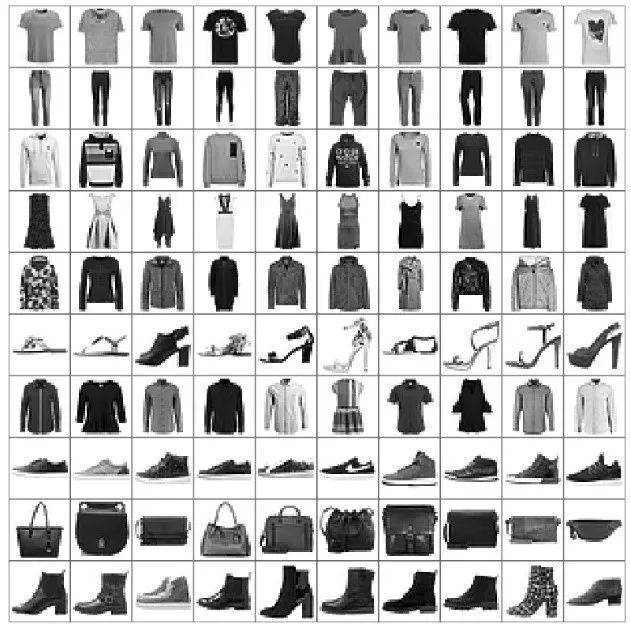▍图 1 Fashion MNIST 数据集

# 加载Fashion MNIST图片数据集(x_train, y_train), (x_test, y_test) = keras.datasets.fashion_mnist.load_data()# 归一化x_train, x_test = x_train.astype(np.float32) / 255., x_test.astype(np.float32) / 255.# 只需要通过图片数据即可构建数据集对象，不需要标签train_db = tf.data.Dataset.from_tensor_slices(x_train)train_db = train_db.shuffle(batchsz * 5).batch(batchsz)# 构建测试集对象test_db = tf.data.Dataset.from_tensor_slices(x_test)test_db = test_db.batch(batchsz)

## 2）利用编码器降维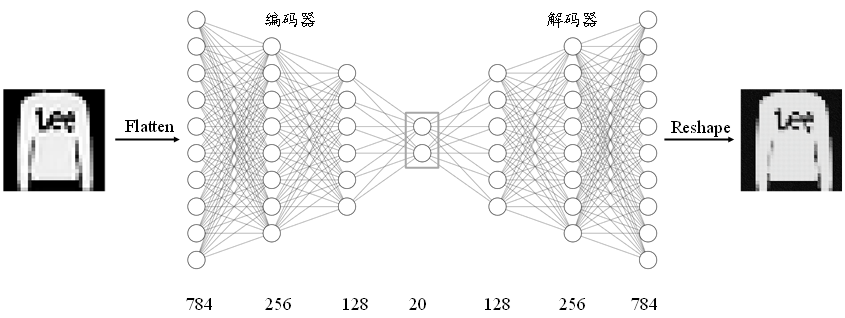▍图 2 Fashion MNIST 自编码器网络结构

# 创建Encoders网络，实现在自编码器类的初始化函数中        self.encoder = Sequential([            layers.Dense(256, activation=tf.nn.relu),            layers.Dense(128, activation=tf.nn.relu),            layers.Dense(h_dim)        ])

## 3）创建解码器

# 创建Decoders网络        self.decoder = Sequential([            layers.Dense(128, activation=tf.nn.relu),            layers.Dense(256, activation=tf.nn.relu),            layers.Dense(784)        ])

## 4）自编码器

class AE(keras.Model):    # 自编码器模型类，包含了Encoder和Decoder2个子网络    def __init__(self):        super(AE, self).__init__()        # 创建Encoders网络        self.encoder = Sequential([            layers.Dense(256, activation=tf.nn.relu),            layers.Dense(128, activation=tf.nn.relu),            layers.Dense(h_dim)        ])        # 创建Decoders网络        self.decoder = Sequential([            layers.Dense(128, activation=tf.nn.relu),            layers.Dense(256, activation=tf.nn.relu),            layers.Dense(784)        ])

def call(self, inputs, training=None):        # 前向传播函数        # 编码获得隐藏向量h,[b, 784] => [b, 20]        h = self.encoder(inputs)        # 解码获得重建图片，[b, 20] => [b, 784]        x_hat = self.decoder(h)        return x_hat

## 5）网络训练

# 创建网络对象model = AE()# 指定输入大小model.build(input_shape=(4, 784))# 打印网络信息model.summary()# 创建优化器，并设置学习率optimizer = optimizers.Adam(lr=lr)

for epoch in range(100): # 训练100个Epoch    for step, x in enumerate(train_db): # 遍历训练集        # 打平，[b, 28, 28] => [b, 784]        x = tf.reshape(x, [-1, 784])        # 构建梯度记录器        with tf.GradientTape() as tape:            # 前向计算获得重建的图片            x_rec_logits = model(x)            # 计算重建图片与输入之间的损失函数            rec_loss = tf.nn.sigmoid_cross_entropy_with_logits(labels=x, logits=x_rec_logits)            # 计算均值            rec_loss = tf.reduce_mean(rec_loss)        # 自动求导，包含了2个子网络的梯度        grads = tape.gradient(rec_loss, model.trainable_variables)        # 自动更新，同时更新2个子网络        optimizer.apply_gradients(zip(grads, model.trainable_variables))        if step % 100 ==0:            # 间隔性打印训练误差            print(epoch, step, float(rec_loss))

## 6）图片重建

 # 重建图片，从测试集采样一批图片    x = next(iter(test_db))    logits = model(tf.reshape(x, [-1, 784])) # 打平并送入自编码器    x_hat = tf.sigmoid(logits) # 将输出转换为像素值，使用sigmoid函数    # 恢复为28x28,[b, 784] => [b, 28, 28]    x_hat = tf.reshape(x_hat, [-1, 28, 28])    # 输入的前50张+重建的前50张图片合并，[b, 28, 28] => [2b, 28, 28]    x_concat = tf.concat([x[:50], x_hat[:50]], axis=0)     x_concat = x_concat.numpy() * 255. # 恢复为0~255范围    x_concat = x_concat.astype(np.uint8)  # 转换为整型save_images(x_concat, 'ae_images/rec_epoch_%d.png'%epoch)  # 保存图片

# 02、运行结果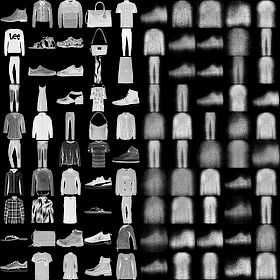▍图 3 第 1 个 Epoch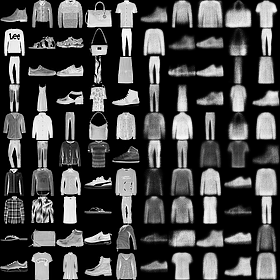▍图 4 第 10 个 Epoch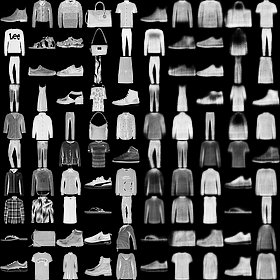▍图 5 第 100 个 Epoch

def save_images(imgs, name):    # 创建280x280大小图片阵列    new_im = Image.new('L', (280, 280))    index = 0    for i in range(0, 280, 28): # 10行图片阵列        for j in range(0, 280, 28): # 10列图片阵列            im = imgs[index]            im = Image.fromarray(im, mode='L')            new_im.paste(im, (i, j)) # 写入对应位置            index += 1    # 保存图片阵列    new_im.save(name)

# 3、完整代码

import  osimport  tensorflow as tfimport  numpy as npfrom    tensorflow import kerasfrom    tensorflow.keras import Sequential, layersfrom    PIL import Imagefrom    matplotlib import pyplot as plttf.random.set_seed(22)np.random.seed(22)os.environ['TF_CPP_MIN_LOG_LEVEL'] = '2'assert tf.__version__.startswith('2.')def save_images(imgs, name):    new_im = Image.new('L', (280, 280))    index = 0    for i in range(0, 280, 28):        for j in range(0, 280, 28):            im = imgs[index]            im = Image.fromarray(im, mode='L')            new_im.paste(im, (i, j))            index += 1    new_im.save(name)h_dim = 20batchsz = 512lr = 1e-3(x_train, y_train), (x_test, y_test) = keras.datasets.fashion_mnist.load_data()x_train, x_test = x_train.astype(np.float32) / 255., x_test.astype(np.float32) / 255.# we do not need labeltrain_db = tf.data.Dataset.from_tensor_slices(x_train)train_db = train_db.shuffle(batchsz * 5).batch(batchsz)test_db = tf.data.Dataset.from_tensor_slices(x_test)test_db = test_db.batch(batchsz)print(x_train.shape, y_train.shape)print(x_test.shape, y_test.shape)class AE(keras.Model):    def __init__(self):        super(AE, self).__init__()        # Encoders        self.encoder = Sequential([            layers.Dense(256, activation=tf.nn.relu),            layers.Dense(128, activation=tf.nn.relu),            layers.Dense(h_dim)        ])        # Decoders        self.decoder = Sequential([            layers.Dense(128, activation=tf.nn.relu),            layers.Dense(256, activation=tf.nn.relu),            layers.Dense(784)        ])    def call(self, inputs, training=None):        # [b, 784] => [b, 10]        h = self.encoder(inputs)        # [b, 10] => [b, 784]        x_hat = self.decoder(h)        return x_hatmodel = AE()model.build(input_shape=(None, 784))model.summary()optimizer = tf.optimizers.Adam(lr=lr)for epoch in range(100):    for step, x in enumerate(train_db):        #[b, 28, 28] => [b, 784]        x = tf.reshape(x, [-1, 784])        with tf.GradientTape() as tape:            x_rec_logits = model(x)            rec_loss = tf.losses.binary_crossentropy(x, x_rec_logits, from_logits=True)            rec_loss = tf.reduce_mean(rec_loss)        grads = tape.gradient(rec_loss, model.trainable_variables)        optimizer.apply_gradients(zip(grads, model.trainable_variables))        if step % 100 ==0:            print(epoch, step, float(rec_loss))        # evaluation        x = next(iter(test_db))        logits = model(tf.reshape(x, [-1, 784]))        x_hat = tf.sigmoid(logits)        # [b, 784] => [b, 28, 28]        x_hat = tf.reshape(x_hat, [-1, 28, 28])        # [b, 28, 28] => [2b, 28, 28]        x_concat = tf.concat([x, x_hat], axis=0)        x_concat = x_hat        x_concat = x_concat.numpy() * 255.        x_concat = x_concat.astype(np.uint8)        save_images(x_concat, 'ae_images/rec_epoch_%d.png'%epoch)### TiAmo

CSDN全栈领域优质创作者，万粉博主；阿里云专家博主、星级博主、技术博主、阿里云问答官，阿里云MVP；华为云享专家；华为Iot专家；亚马逊人工智能自动驾驶（大众组）吉尼斯世界纪录获得者

## 评论